# MCQs on Digital Electronics

##### Page 34 of 35. Go to page 1 2 3 4 5 6 7 8 9 10 11 12 13 14 15 16 17 18 19 20 21 22 23 24 25 26 27 28 29 30 31 32 33 34 35
01․ Which of the following is 2’s complement of +12?
00001000
00000011
01110011
00001100

In 2’s complement representation a positive number is represented as binary representation of the number itself. i.e. the 2’s complement representation of +12 is 00001100

02․ 11001, 1001 and 111001 correspond to the 2’scomplement representation of the following set of numbers
25, 9 and 57 respectively
-6, -6 and -6 respectively
-7, -7 and -7 respectively
-25, -9 and -57 respectively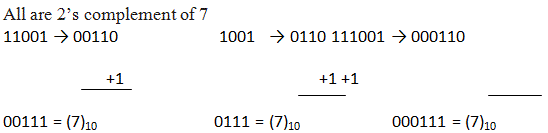03․ Which one of the following is 2’s complement of -68?
11000100
01111100
10111100
00111100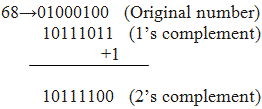04․ A signed integer has been stored in a byte using 2’scomplement format. We wish to store the same integer in 16-bit word. We should copy the original byte to the less significant byte of the word and fill the more significant byte with
0
1
Equal to the MSB of the original byte
Complement of the MSB of the original byte

Let us see this case with the help of an example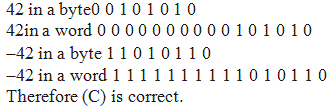05․ The number A = 01110000 and B = 10101111 are in 2’s complement form .Addition of above two number is
00011111
10101111
11100000
011100001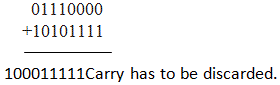06․ The base of the number system for the addition operation 24 + 14 = 41 to be true is
8
7
6
5

Let the base is X, then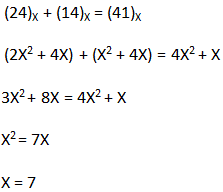07․ A system has following negative numbers stored in binary form as shown. The wrongly stored number is
-32 as 11100000
-37 as 11011011
-48 as 11101000
-89 as 10100111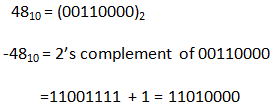08․ Which one of the following is correct sequence of the numbers represented in the series(2)3, (10)4, (11)5, (14)6, (22)7,…………….
2, 3, 4, 5, 6, ….
2, 4, 6, 8, 10, …..
2, 4, 6, 10, 12, …
2, 4, 6, 10, 16……..

Converting into decimal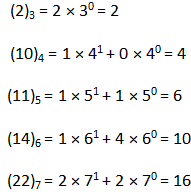09․ X = 01110 and Y = 11001 are two 5-bit binary numbers represented in 2’s complement format. The sum of X and Y in 2’s complement format using 6 bits is
100111
001000
0001111
101001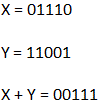Carry is discarded in the addition of numbers represented in 2’s complement form. X + Y in 6 bits are 000111.

10․ A computer has a word length of 8 bits (including sign). It is required to determine the range of integers that can be stored in the computer. If 2’s complement is used to represent the numbers, then the range will be
-256 to 256
-256 to 255
-128 to 128
-128 to 127

For a word length of N, the range of 2,s complement numbers that can be represented is - 2N - 1 to 2N - 1 - 1. So, for a word length of 8, the range is - 27 to 27 - 1 i.e. - 128 to 127

<<<32333435>>>